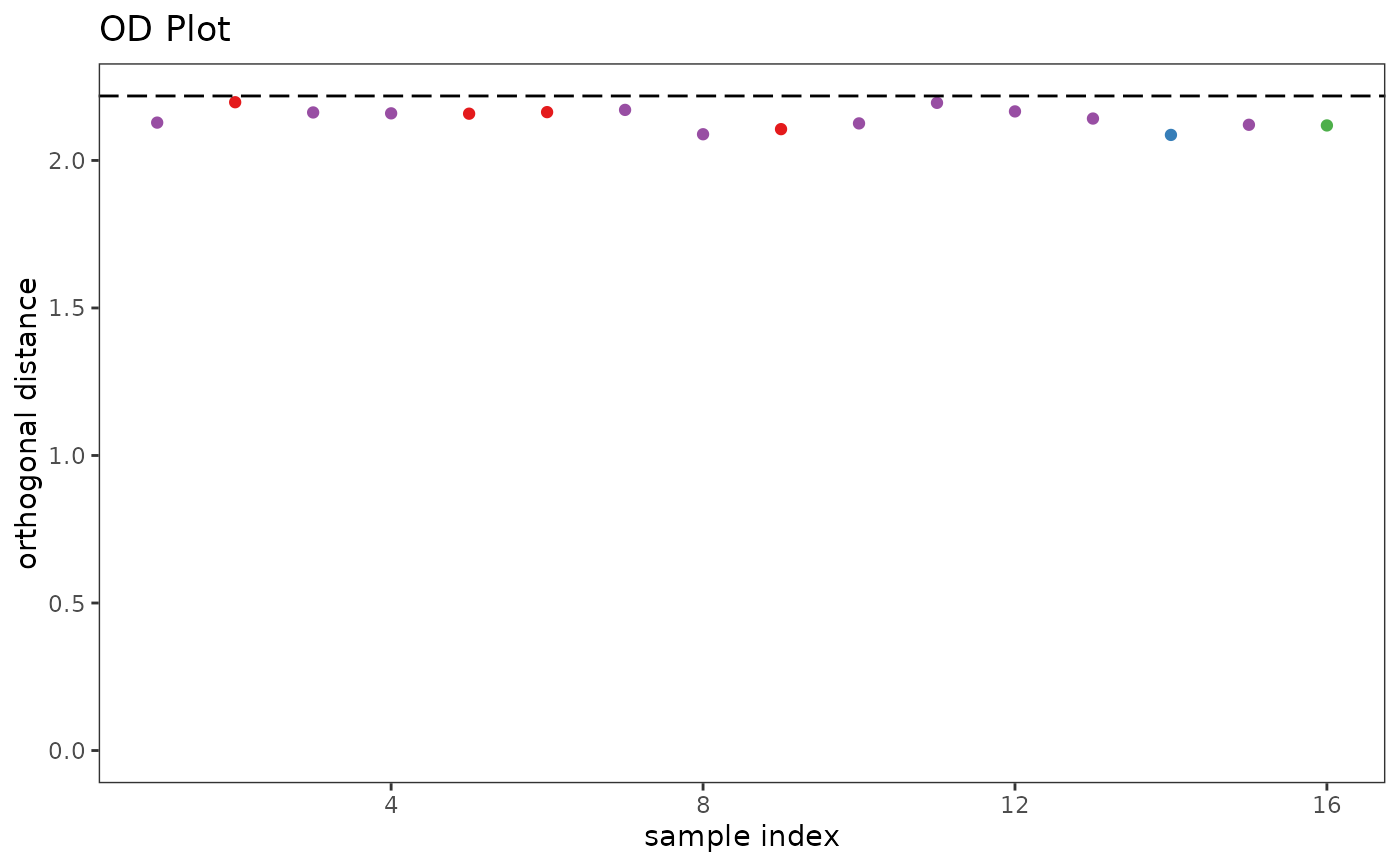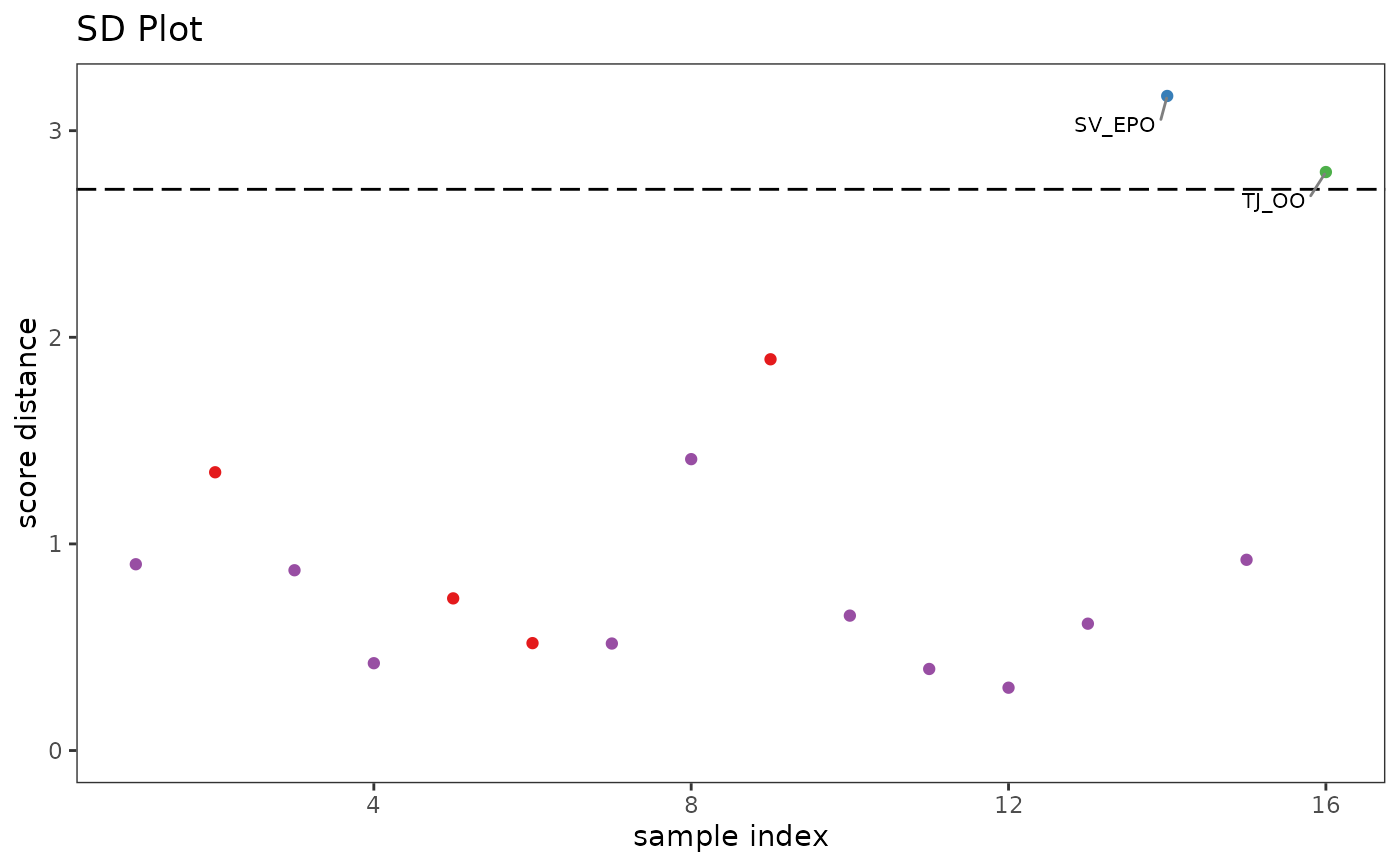A function to carry diagnostics on the PCA results for a Spectra object. Basically a wrapper to Filzmoser's pcaDiagplot which colors everything according to the scheme stored in the Spectra object. Works with PCA results of either class prcomp or class princomp. Works with either classical or robust PCA results.

pcaDiag(
spectra,
pca,
pcs = 3,
quantile = 0.975,
plot = c("OD", "SD"),
use.sym = FALSE,
...
)

## Arguments

spectra

An object of S3 class Spectra.

pca

An object of class prcomp modified to include a character string (\$method) describing the pre-processing carried out and the type of PCA performed.

pcs

As per pcaDiagplot. The number of principal components to include.

quantile

As per pcaDiagplot. The significance criteria to use as a cutoff.

plot

A character string, indicating whether to plot the score distances or orthogonal distances, or both. Options are c("OD", "SD").

use.sym

logical; if true, the color scheme is change to black and symbols are used for plotting.

...

Parameters to be passed to the plotting routines. Applies to base graphics only.

## Value

The returned value depends on the graphics option selected (see GraphicsOptions).

base:

A data frame or list containing the data plotted. Assign the value and run str() or names() on it to see what it contains. Side effect is a plot.

ggplot2:

The plot is displayed, and a ggplot2 plot object is returned if the value is assigned. The plot can be modified in the usual ggplot2 manner. If you want the plotted values, you can access them via the base graphics mode.

## Details

If both plots are desired, the output should be directed to a file rather than the screen. Otherwise, the 2nd plot overwrites the 1st in the active graphics window. Alternatively, just call the function twice, once specifying OD and once specifying SD.

pcaDiagplot in package chemometrics for the underlying function. Additional documentation at https://bryanhanson.github.io/ChemoSpec/

## Author

Bryan A. Hanson (DePauw University), Tejasvi Gupta.

## Examples

# This example assumes the graphics output is set to ggplot2 (see ?GraphicsOptions).
library("ggplot2")
data(SrE.IR)
pca <- c_pcaSpectra(SrE.IR, choice = "noscale")
p1 <- pcaDiag(SrE.IR, pca, pcs = 2, plot = "OD") + ggtitle("OD Plot")
p1p2 <- pcaDiag(SrE.IR, pca, pcs = 2, plot = "SD") + ggtitle("SD Plot")
p2